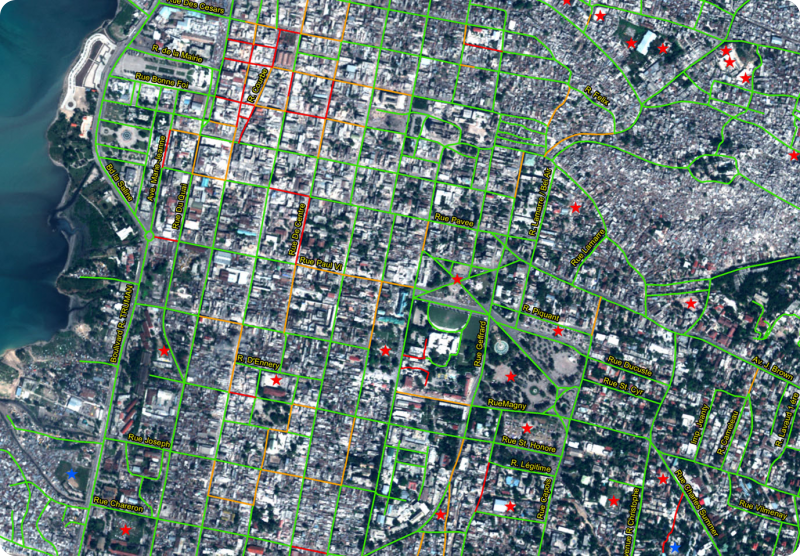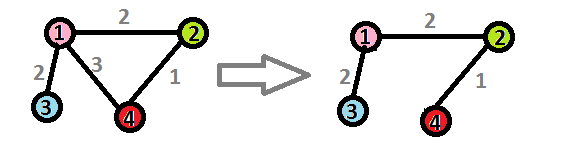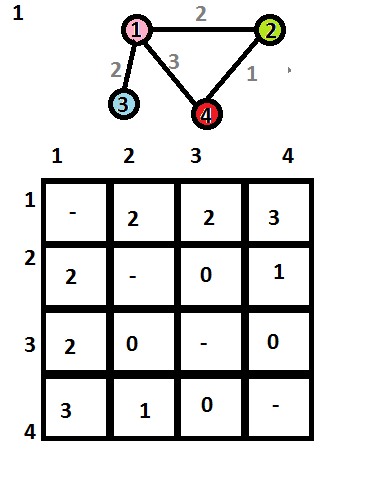# Help the engineerAn engineer working for a telecom company wants to design a cable network in a small nation. He can only bury the cables along main roads. Some paths are more expensive than others. It turns out that a previous employee of the company had already layed a network that is not efficient. In order to minimize costs, our engineer wants to optimize the network by removing some edges while keeping all points on the network connected.

For example, the network below can be optimized by removing the edge from $1$ to $4$:This saves a cost of $3$.

The engineer is given this larger network to optimize. What is the total cost saved?

Details and assumtions

• The network is represented in the text file is represented in an adjacency matrix representation. Basically, the cost from node $x$ to node $y$ is represented in the matrix $A$ as $A_{xy}$(column $x$, row$y$). $A_{xy}=0$ if a path doesnt exist from $x$ to $y$.

• The example network in the picture above has the following adjacency matrix representation:×